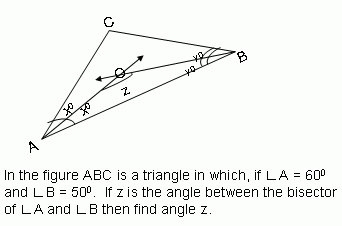Email us to get an instant 20% discount on highly effective K-12 Math & English kwizNET Programs!

#### Online Quiz (WorksheetABCD)

Questions Per Quiz = 2 4 6 8 10

### Geometry2.28 Triangles Review Test

 Q 1:115 degrees125 degrees110 degrees120 degrees Q 2: Find the measure of each of the exterior angle of a regular polygon of 4 sides.90 degrees80 degrees100 degrees Q 3: Find angle EAB75o45o60o Q 4: The sum of the interior angles of a polygon of 12 sides is ____ degrees.Answer: Q 5: The sides of a triangle are 50cm, 80cm, 100cm, check if this is a right angled triangle.noyes Q 6: The ______ of a triangle is the point of concurrence of the perpendicular bisectors of the sides of the triangle.circumcentrecircumcirclecircumradius Q 7:60 degrees115 degrees55 degrees Q 8: InABC, ĐA = 100°, ĐB = 50°, find ĐC.30°35°60°70° Question 9: This question is available to subscribers only! Question 10: This question is available to subscribers only!